Author: Oscar Cronquist Article last updated on May 18, 2022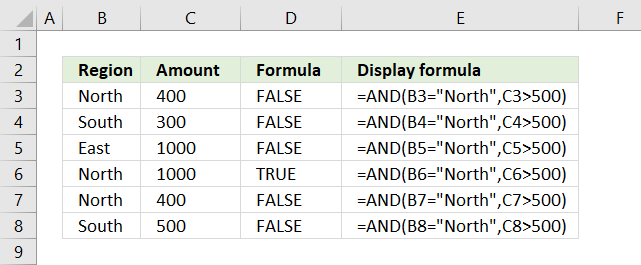The image above demonstrates the AND function with two logical expressions. If the value in column B is equal to "North" and the value on the same row in column C is above 500 the AND function returns TRUE. These conditions are only met on row 6.

Formula in cell D3:

=AND(B3="North", C3>500)

## 1. Excel function syntax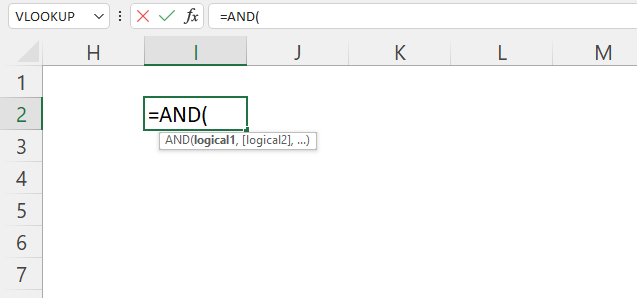AND(logical1, [logical2], ...)

The AND function allows you to perform a logical test in each argument and if all arguments return TRUE the AND function returns TRUE. If at least one argument returns FALSE the AND function returns FALSE.

## 2. Argumentslogical1 Required. A logical expression or a function that returns a number. [logical2] Optional. Also a logical expression or a function that returns a number. You can have up to 254 arguments.

## 3. How to use numerical values in the AND function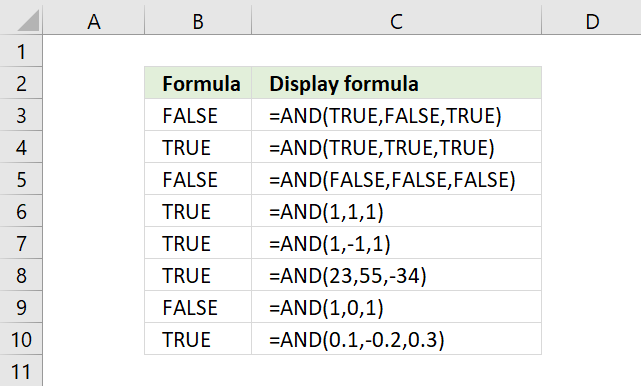The AND function arguments can result in TRUE or FALSE, however, it also treats all numbers, both positive and negative, as TRUE.

The exception to that is 0 (zero) which is treated the same as FALSE.

## 4. How to use an array with the AND function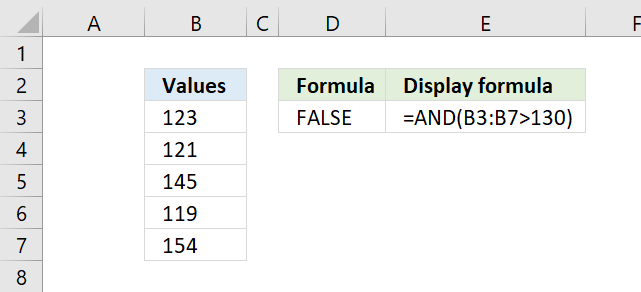The AND function also allows you to not only compare a single cell value but also an entire cell range.

Formula in cell E3:

=AND(B3:B7>130)

To enter the formula above as an array formula, type the formula in a cell. Press and hold CTRL + SHIFT keys simultaneously, then press Enter once. Release all keys. The formula is now enclosed with curly brackets, they indicate you successfully entered the formula as an array formula. Don't enter the curly brackets yourself.

### 4.1 Explaining formula in cell E3

#### Step 1 - Check if array values are larger than the condition

The larger than character is a logical operator that returns boolean value TRUE if the value is larger than a condition and FALSE if not.

B3:B7>130

becomes

{123; 121; 145; 119; 154}>130

An array may contain values arranged in a single column/row or in a 2D array meaning multiple columns and rows.

{123; 121; 145; 119; 154}>130

and returns

{FALSE; FALSE; TRUE; FALSE; TRUE}

#### Step 2 - Evaluate AND function

The formula in cell D3 checks if each value in cell range B3:B7 is larger than 130. Three values are not larger than 130 so the AND function returns FALSE.

AND(B3:B7>130)

becomes

AND({FALSE; FALSE; TRUE; FALSE; TRUE})

and returns FALSE. All booleans values must be TRUE for the AND function to return TRUE.

### 4.2 How to use multiple arrays with the AND function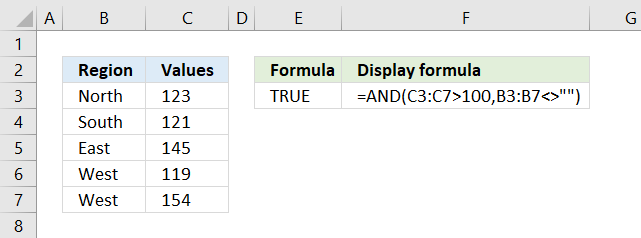The array formula above in cell E3 has two arguments containing a logical expression in each. The first argument checks if values in cell range C3:C7 are larger than 100.

Formula in cell E3:

=AND(C3:C7>100, B3:B7<>"")

#### Step 1 - First condition

C3:C7>100

becomes

{123; 121; 145; 119; 154}>100

and returns

{TRUE; TRUE; TRUE; TRUE; TRUE}

#### Step 2 - Second condition

The second argument checks if values in cell range B3:B7 are not empty.

B3:B7<>""

becomes

{"North";"South";"East";"West";"West"}<>""

and returns

{TRUE; TRUE; TRUE; TRUE; TRUE}.

#### Step 3 - Evaluate AND function

All values are TRUE so the AND function returns TRUE.

AND(C3:C7>100,B3:B7<>"")

becomes

AND({TRUE;TRUE;TRUE;TRUE;TRUE},{TRUE; TRUE; TRUE; TRUE; TRUE}) and returns TRUE.

There are two separate arrays in the AND function, however, it doesn't matter. All values must be TRUE or its numerical equivalent to return TRUE.

## 5. AND function in IF function example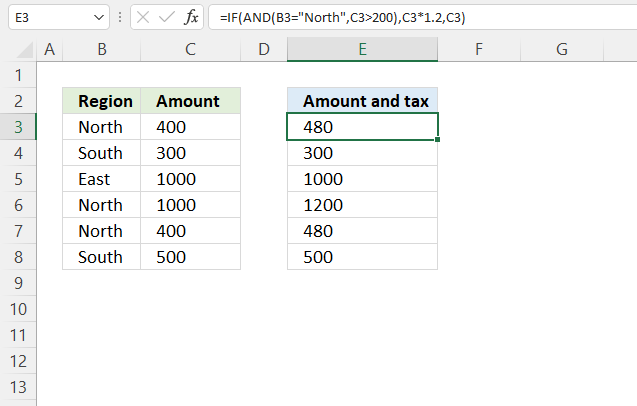The image above demonstrates a formula that combines the AND function and the IF function. If the "Region" is equal to "North" and the "Amount" on the same row is above 200 then multiply with 1.2.

If not return the amount only.

Formula in cell E3:

=IF(AND(B3="North", C3>200), 1.2*C3, C3)

### 5.1 Explaining formula in cell E3

#### Step 1 - First condition

The equal sign is a logical operator that returns boolean value TRUE if a value matches another value. It returns FALSE if not.

B3="North"

becomes

"North"="North"

and returns TRUE.

#### Step 2 - Second condition

The larger than sign is a logical operator that returns boolean value TRUE if a value is larger than another value. It returns FALSE if not.

C3>200

becomes

400>200

and returns TRUE.

#### Step 3 - Evaluate AND function

The AND function returns TRUE if all arguments evaluates to TRUE.

AND(B3="North", C3>200)

becomes

AND(TRUE, TRUE)

#### Step 4 - Calculate IF function

The IF function returns one value if the logical test is TRUE and another value if the logical test is FALSE.

IF(logical_test, [value_if_true], [value_if_false])

IF(AND(B3="North", C3>200), 1.2*C3, C3)

becomes

IF(TRUE, 1.2*C3, C3)

becomes

IF(TRUE, 1.2*400, C3)

and returns 480.

## 6. AND function with text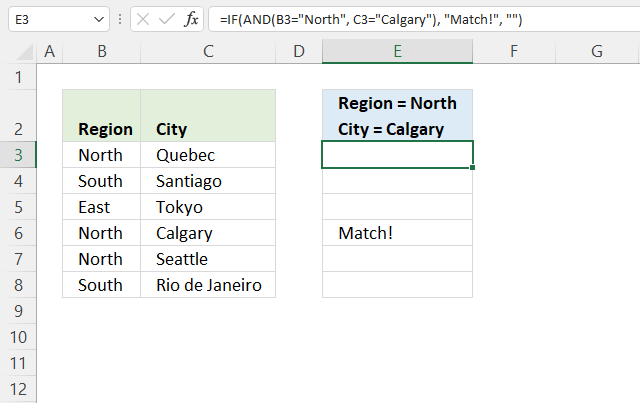The formula in cell E3 checks if cell B3 is equal to a given text condition and cell C3 is equal to another given text condition, both conditions must be met to returns "Match!".

Cell E6 returns "Match!", both conditions are met.

Formula in cell E3:

=IF(AND(B3="North", C3="Calgary"), "Match!", "")

### 6.1 Explaining formula in cell E3

#### Step 1 - First condition

The equal sign lets you compare value to value, it is a logical operator and returns a boolean value TRUE or FALSE.

B3="North"

becomes

"North" = "North"

and returns TRUE.

#### Step 2 - Second condition

C3="Calgary")

becomes

"Quebec"="Calgary")

and returns FALSE.

#### Step 3 - Evaluate AND function

The AND function returns TRUE if all arguments are TRUE.

AND(B3="North", C3="Calgary")

becomes

AND(TRUE, FALSE)

and returns FALSE.

#### Step 4 - Evaluate IF function

The IF function returns one value if the logical test is TRUE and another value if the logical test is FALSE.

IF(logical_test, [value_if_true], [value_if_false])

IF(AND(B3="North", C3="Calgary"), "Match!", "")

becomes

IF(FALSE, "Match!", "")

and returns "".

## 7. AND function Conditional formatting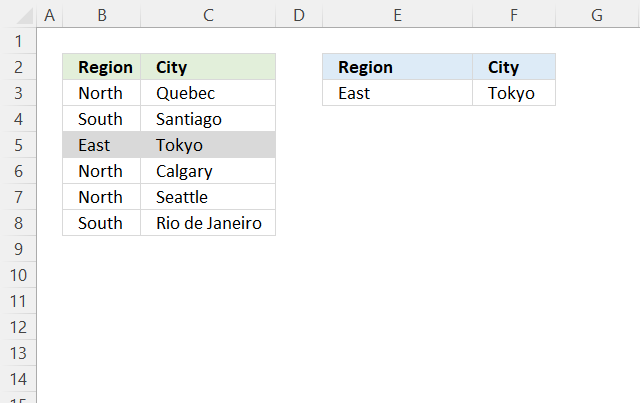The image above shows two cells highlighted by the Conditional formatting formula applied to cell range B3:C8. The conditions are specified in cells E3:F3 respectively.

Both values in columns B and C are highlighted if they match the conditions, the example above shows cell B5:C5 highlighted, they match cells E3 and F3.

Conditional formatting formula in cell B3:

=AND(\$E\$3=\$B3, \$F\$3=\$C3)

### 7.1 How to apply Conditional Formatting?

1. Select cell range B3:C8.
2. Go to tab "Home" on the ribbon.
3. Press with the mouse on the "Conditional Formatting" button.
4. Press on "New Rule...". A popup menu appears.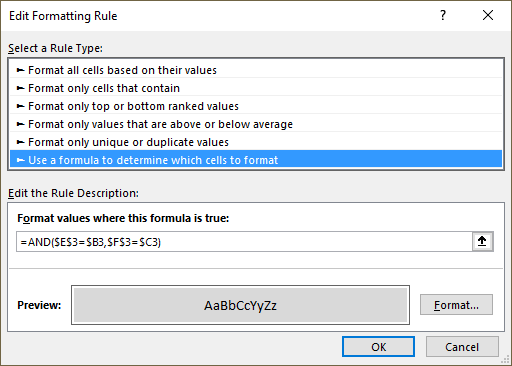5. Select "Use a formula to determine which cells to format".
6. Paste the formula above to "Format values where this is true:".
7. Press the OK button.

### 7.2 Explaining conditional formatting formula in cell B3

#### Step 1 - First condition

The equal sign lets you compare value to value, it is a logical operator and returns a boolean value TRUE or FALSE.

The dollar sign lets you lock the column or the row number. When the Conditional Formatting tool moves from cell to cell in cell range B3:C8 cell \$B3 stays in column B, however, it may freely move to rows below.

\$E\$3=\$B3

becomes

"East" = "North"

and returns FALSE.

#### Step 2 - Second condition

Reference \$C3 is locked to column C.

\$F\$3=\$C3

becomes

"Tokyo"="Santiago"

and returns FALSE.

#### Step 3 - Evaluate AND function

The AND function returns TRUE if all arguments are TRUE.

AND(\$E\$3=\$B3, \$F\$3=\$C3)

becomes

AND(TRUE, TRUE)

and returns TRUE.